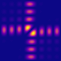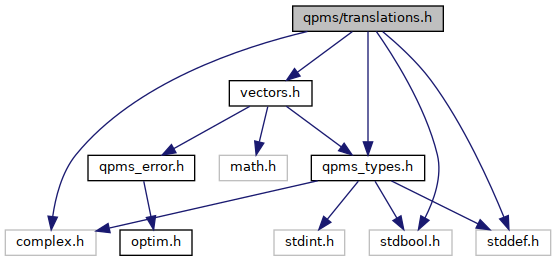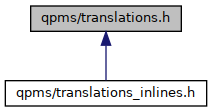QPMS Electromagnetic multiple scattering library and toolkit.
translations.h File Reference

VSWF translation operator. More...

#include "vectors.h"
#include "qpms_types.h"
#include <complex.h>
#include <stdbool.h>
#include <stddef.h>
Include dependency graph for translations.h:This graph shows which files directly or indirectly include this file:Go to the source code of this file.

## Data Structures

struct  qpms_trans_calculator
Structure holding the constant factors in normalisation operators. More...

## Typedefs

typedef struct qpms_trans_calculator qpms_trans_calculator
Structure holding the constant factors in normalisation operators. More...

## Functions

complex double qpms_trans_single_A (qpms_normalisation_t norm, qpms_m_t m, qpms_l_t n, qpms_m_t mu, qpms_l_t nu, csph_t kdlj, bool r_ge_d, qpms_bessel_t J)

complex double qpms_trans_single_B (qpms_normalisation_t norm, qpms_m_t m, qpms_l_t n, qpms_m_t mu, qpms_l_t nu, csph_t kdlj, bool r_ge_d, qpms_bessel_t J)

qpms_trans_calculatorqpms_trans_calculator_init (qpms_l_t lMax, qpms_normalisation_t nt)
Initialise a qpms_trans_calculator_t instance for a given convention and truncation degree. More...

void qpms_trans_calculator_free (qpms_trans_calculator *)
Destructor for qpms_trans_calculator_t.

complex double qpms_trans_calculator_get_A (const qpms_trans_calculator *c, qpms_m_t m, qpms_l_t n, qpms_m_t mu, qpms_l_t nu, csph_t kdlj, bool r_ge_d, qpms_bessel_t J)

complex double qpms_trans_calculator_get_B (const qpms_trans_calculator *c, qpms_m_t m, qpms_l_t n, qpms_m_t mu, qpms_l_t nu, csph_t kdlj, bool r_ge_d, qpms_bessel_t J)

int qpms_trans_calculator_get_AB_p (const qpms_trans_calculator *c, complex double *Adest, complex double *Bdest, qpms_m_t m, qpms_l_t n, qpms_m_t mu, qpms_l_t nu, csph_t kdlj, bool r_ge_d, qpms_bessel_t J)

int qpms_trans_calculator_get_AB_arrays (const qpms_trans_calculator *c, complex double *Adest, complex double *Bdest, size_t deststride, size_t srcstride, csph_t kdlj, bool r_ge_d, qpms_bessel_t J)

complex double qpms_trans_calculator_get_A_ext (const qpms_trans_calculator *c, int m, int n, int mu, int nu, complex double kdlj_r, double kdlj_th, double kdlj_phi, int r_ge_d, int J)

complex double qpms_trans_calculator_get_B_ext (const qpms_trans_calculator *c, int m, int n, int mu, int nu, complex double kdlj_r, double kdlj_th, double kdlj_phi, int r_ge_d, int J)

int qpms_trans_calculator_get_AB_p_ext (const qpms_trans_calculator *c, complex double *Adest, complex double *Bdest, int m, int n, int mu, int nu, complex double kdlj_r, double kdlj_th, double kdlj_phi, int r_ge_d, int J)

int qpms_trans_calculator_get_AB_arrays_ext (const qpms_trans_calculator *c, complex double *Adest, complex double *Bdest, size_t deststride, size_t srcstride, complex double kdlj_r, double kdlj_theta, double kdlj_phi, int r_ge_d, int J)

qpms_errno_t qpms_trans_calculator_get_trans_array (const qpms_trans_calculator *c, complex double *target, const qpms_vswf_set_spec_t *destspec, size_t deststride, const qpms_vswf_set_spec_t *srcspec, size_t srcstride, csph_t kdlj, bool r_ge_d, qpms_bessel_t J)

qpms_errno_t qpms_trans_calculator_get_trans_array_lc3p (const qpms_trans_calculator *c, complex double *target, const qpms_vswf_set_spec_t *destspec, size_t deststride, const qpms_vswf_set_spec_t *srcspec, size_t srcstride, complex double k, cart3_t destpos, cart3_t srcpos, qpms_bessel_t J)

## Detailed Description

VSWF translation operator.

### Argument conventions

A single wave with indices mu, nu is re-expanded at kdlj into waves with indices m, n, i.e. in the following functions, the first arguments over which one sums (multiplied by the waves with new origin).

HOWEVER, this means that if a field has an expansion with coeffs a(mu, nu) at the original origin, with the basis at the new origin, the coeffs will be a(m, n) = \sum_{mu,nu} A(m, n, mu, nu) a(mu, nu).

With qpms_trans_calculator_get_AB_arrays_buf (and other functions from AB_arrays family), one can choose the stride. And it seems that the former stride argument (now called destride) and the latter (now called srcstride) are connected to (m,n) and (mu,nu) indices, respectively. Seems consistent.

#### r_ge_d argument:

If r_ge_d == true, the translation coefficients are calculated using regular bessel functions, regardless of what J argument is.

## ◆ qpms_trans_calculator

 typedef struct qpms_trans_calculator qpms_trans_calculator

Structure holding the constant factors in normalisation operators.

The VSWF translation operator elements are rather complicated linear combinations of Bessel functions and spherical harmonics. The preceding factors are rather complicated but need to be calculated (for a single normalisation convention) only once and then recycled during the operator evaluation for different translations.

This structure is initialised with qpms_trans_calculator_t() and holds all the constant factors up to a truncation degree lMax.

The destructor function is qpms_trans_calculator_free().

If Ewald sums are enabled at build, it also holds the constant factors useful for lattice sums of translation operator.

## ◆ qpms_trans_calculator_get_trans_array()

 qpms_errno_t qpms_trans_calculator_get_trans_array ( const qpms_trans_calculator * c, complex double * target, const qpms_vswf_set_spec_t * destspec, size_t deststride, const qpms_vswf_set_spec_t * srcspec, size_t srcstride, csph_t kdlj, bool r_ge_d, qpms_bessel_t J )
Parameters
 destspec Must be destspec->lMax <= c-> lMax && destspec->norm == c->norm. srcspec Must be srcspec->lMax <= c-> lMax && srcspec->norm == c->norm.

## ◆ qpms_trans_calculator_get_trans_array_lc3p()

 qpms_errno_t qpms_trans_calculator_get_trans_array_lc3p ( const qpms_trans_calculator * c, complex double * target, const qpms_vswf_set_spec_t * destspec, size_t deststride, const qpms_vswf_set_spec_t * srcspec, size_t srcstride, complex double k, cart3_t destpos, cart3_t srcpos, qpms_bessel_t J )

Version with k and cartesian particle positions and with automatic r_ge_d = false.

Parameters
 destspec Must be destspec->lMax <= c-> lMax && destspec->norm == c->norm. srcspec Must be srcspec->lMax <= c-> lMax && srcspec->norm == c->norm. J Workspace has to be at least 2 * c->neleme**2 long

## ◆ qpms_trans_calculator_init()

 qpms_trans_calculator* qpms_trans_calculator_init ( qpms_l_t lMax, qpms_normalisation_t nt )

Initialise a qpms_trans_calculator_t instance for a given convention and truncation degree.

Parameters
 lMax Truncation degree.

## ◆ qpms_trans_single_A()

 complex double qpms_trans_single_A ( qpms_normalisation_t norm, qpms_m_t m, qpms_l_t n, qpms_m_t mu, qpms_l_t nu, csph_t kdlj, bool r_ge_d, qpms_bessel_t J )

N<-N type coefficients w.r.t. Kristensson's convention. Csphase has been already taken into acct ^^^.

## ◆ qpms_trans_single_B()

 complex double qpms_trans_single_B ( qpms_normalisation_t norm, qpms_m_t m, qpms_l_t n, qpms_m_t mu, qpms_l_t nu, csph_t kdlj, bool r_ge_d, qpms_bessel_t J )

N<-M type coefficients w.r.t. Kristensson's convention. Csphase has been already taken into acct ^^^.